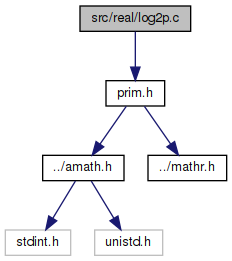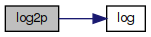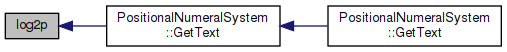amath  1.8.5 Simple command line calculator
log2p.c File Reference
`#include "prim.h"`
Include dependency graph for log2p.c:Go to the source code of this file.

## Functions

double log2p (double x, double y)

## ◆ log2p()

 double log2p ( double x, double y )

Definition at line 32 of file log2p.c.

References log().

Referenced by PositionalNumeralSystem::GetText().

33 {
34  return y != 1.0
35  ? log(y) / log(x)
36  : 0.0;
37 }
double log(double x)
Natural logarithm function (base e)
Definition: log.c:109
Here is the call graph for this function:Here is the caller graph for this function: